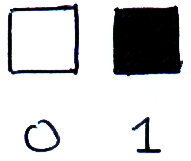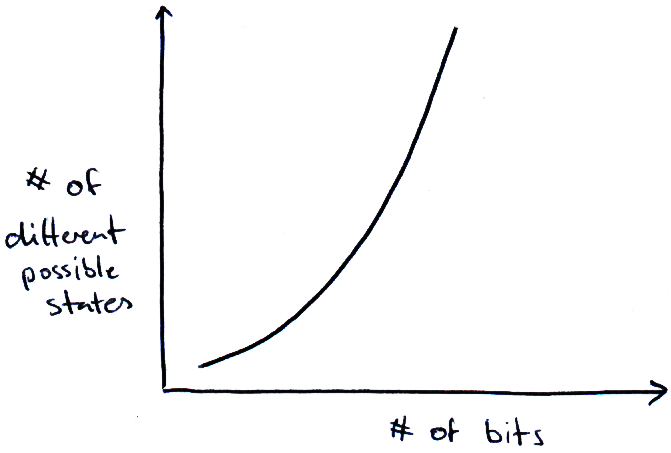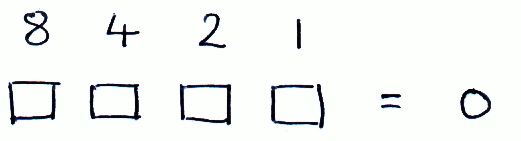# Preliminary Computer Science

## Bits & BinaryThe bit is the most basic carrier of information. It can be in one of two states, 0 or 1, and we can combine bits to represent larger amounts of information. If we have n bits, there are 2n different possible states our bits can be in. We can see that the amount of information that can be stored in our bits grows exponentially with n:We can represent numbers using binary so that the number can be stored in bits:Anything that can be in one of two states can be used as a bit. On early computers, card was used to store bits, at each point along the grid, a hole represents 1 and a lack of hole represents 0:

This card hold 960 bits. In comparison, modern hard drives can contain around 8000000000000 bits (1 Terabyte)!